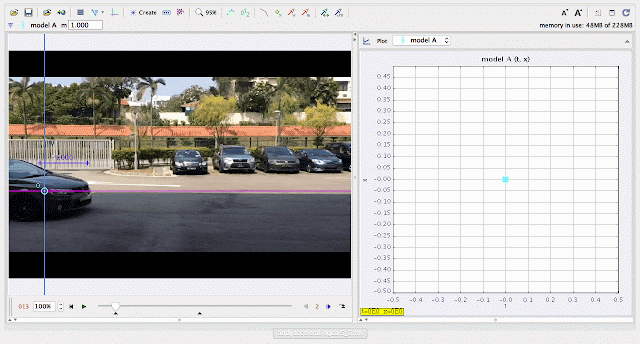{source}
<?phprequire_once JPATH_SITE.'/TTcustom/TT_contentparser.php';//include 'TT_contentparser.php';$$parameters = array( "topicname" => "02_newtonianmechanics_2kinematics", //e.g. 02_newtonianmechanics_2kinematics"modelname" => "andyacceleratingcar5_5"); //e.g. airpuckconstantvelocity.trzecho generateSimHTML($$parameters, "TRZ");
?>
{/source}

### ICT Connection Lessonusing Tracker Model Builder to create theoretic graphs for simplifying Physics concepts. increasing position x^3 motion is illustrated through position x versus time graphusing Tracker Model Builder to create theoretic graphs for simplifying Physics concepts. increasing velocity vx^2 motion is illustrated through position vx versus time graphusing Tracker Model Builder to create theoretic graphs for simplifying Physics concepts. increasing acceleration ax^1 motion is illustrated through position ax versus time graph

end faq

Rating 5.00 (1 Vote)